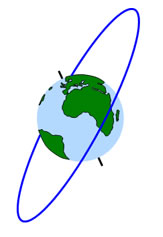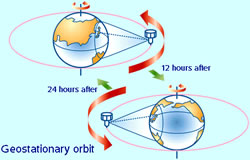# Difference between Geosynchronous Orbit and Geostationary Orbit

##### Key Difference: A geosynchronous orbit is an orbit around a planet which has the same orbital period as the planet’s rotation period.  Geostationary orbit is a kind of geosynchronous orbit but with an addition feature. It remains stationary with respect to a single point on the surface of the planet.

Many people confuse geosynchronous orbit and geostationary orbit. People tend to think that both are the same and often use them as synonyms. However, geostationary orbit is a type of geosynchronous orbit.

To understand these orbits, one must be clear about the concept of an orbit. An orbit is a curved path that is taken by an object around a star, planet, moon, etc. Thus, we can simply define it "as the path determing the motion of a body around the other body". The most common example is our solar system in which planets like Earth, Saturn and Jupiter follow an orbit around the sun.Geosynchronous orbit has the same orbital period as the rotation period of a central body around which the object is following this path. The term is mostly used in context to satellites that move around the Earth.

Earth takes 24 hours to complete one rotation and thus geosynchronous satellites of the Earth also takes 24 hours to complete their orbit. There are many geosynchronous orbits that are present around the Earth.

We all are aware with the fact, that the Earth rotates about an imaginary line that passes through the North and South poles of the planet. This particular line can also be referred to as the axis of rotation. To simplify, keep your thump pointing upward in a thumps up position. The direction of thumb can denote the axis of rotation of the Earth and the direction of fingers can describe the direction of rotation of the Earth. If we want that an object must remain in a fixed location in an orbit with respect to the Earth, then we have to consider a few points like:-

• Earth is rotating.
• Satellite around Earth is also following an orbit path thus, it is also moving.

To make a satellite look constant or fixed with respect to the Earth, the orbit needs to be a circular orbit and that too stationed over the equator. If a satellite will be fixed with these attributes, the movement of the Earth and satellite will be synchronized and the satellite's position will be fixed with respect to a fixed location on the Earth.Features of geostationary orbit around the Earth:-

• This orbit is present at an altitude of approx. 35,786 km in the equatorial plane.
• No tracking equipment is required to send or receive signals from the satellites placed in this orbit.
• Geostationary satellites are used to provide continuous service over a large and wide area.
• These satellites placed in geostationary orbit have trouble monitoring activities near the poles.

From the above explanation, it is clear that a geostationary orbit is a type of geosynchronous orbit. A geosynchronous orbit can be any orbit, like with an elliptical path, that has a period equal to the Earth’s rotational period, whereas a geostationary orbit has to be a circular orbit and that too placed above the equator. However, in case of a satellite, the choice of a particular orbit depends upon its mission. There can be many geosynchronous orbits but only one geostationary orbit with respect to Earth as the central body.

Image Courtesy: halo.wikia.com, newmediastudio.org